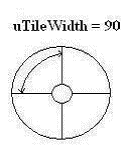﻿ MOSAICTILESINFO | Used Structures | Raster Imaging C API Help

MOSAICTILESINFO

typedef struct tagMOSAICTILESINFO
{
L_UINT uStructSize;
L_UINT uOpacity;
L_UINT uPenWidth;
L_UINT uTileWidth;
L_UINT uTileHeight;
POINT ptCenter;
L_UINT uFlags;
COLORREF crBorderColor;
COLORREF crTilesColor;
}MOSAICTILESINFO, *pMOSAICTILESINFO;

The MOSAICTILESINFO structure provides the information needed for the L_MosaicTilesBitmap function.

 Member Description uStructSize Size of this structure, in bytes. Use the sizeof operator to calculate this value. uOpacity Value that represents the weight, in percent, given to the image color and the border or tile color when determining the resulting color. For example, if uOpacity = 75, then 75% of the image color will be added to 75% of the border (or Tile) color. Final results are clipped if greater than 255. Possible values are from 0 to 100. uPenWidth Value that indicates the width of the pen used to draw tile boundaries, in logical units. Possible values range from 1 to 15. The pen uses the crBorderColor. uTileWidth Value that represents the width of the tile. The meaning of uTileWidth differs according to the flags that are set in the uFlags member. Possible values are: If uFlags contains uTileWidth represents Valid Range MSCT_CART The width of each rectangular tile, in pixels. 1 to the image width, if there is no region. If there is a region, then the range of values is 1 to the region width. MSCT_POLAR The size, in degrees, of the curve in the arc-shaped tiles. That is, the circle around the ptCenter is divided into tiles of uTileWidth degrees, as shown below:1 to 360. The circle can be divided into tiles from 1 degree to 360 degrees. uTileHeight Value that represents the height of the tile. The uTileHeight differs according to the flags that are set in the uFlags member. Possible values are: If uFlags contains uTileHeight represents Valid Range MSCT_CART The height of each rectangular tile, in pixels. 1 to the image height, if there is no region. If there is a region, then the range of values is 1 to the region height. MSCT_POLAR The radial length of each arc-shaped tile, in pixels. 1 to the diagonal of the image, if there is no region. If there is a region, then the range of values is 1 to the diagonal of the region rectangle. ptCenter Point that represents the center of revolution when polar coordinates are used. This parameter is used if MSCT_POLAR is set in uFlags.

 uFlags Flags that indicate whether to use arc-shaped or rectangular-shaped tiles, and what type of shadowing to use, if any. You can use a bit wise OR (|) to specify one flag from each group. The following flags indicate which tile shape should be used: Value Meaning MSCT_CART [0x0000] Draw rectangular tiles. If this flag is used, uTileWidth contains the tile width in pixels, and uTileHeight contains the tile height in pixels. MSCT_POLAR [0x0001] Draw arc-shaped tiles. If this flag used, uTileWidth contains the size of the curve in the tiles in degrees, and uTileHeight contains the radial length of the tiles (in pixels). The following are the flags indicate how to apply the shadow on the tiles, or whether to use shadows: Value Meaning MSCT_FLAT [0x0000] Do not apply a shadow. MSCT_SHADOWRGB [0x0010] Apply colored shadows. MSCT_SHADOWGRAY [0x0020] Apply grayscale shadows.